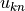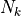# Free energy surfaces with pymbar¶

## Free energy surfces¶

`pymbar` can be used to estimate free energy surfaces using samples from K biased simulations. It is important to note that MBAR itself is not enough to generate a free energy surface. MBAR takes a set of samples from K different states, and can compute the weight that should be given to each sample in in the unbiased state, i.e. the state in which one desires to compute the free energy surface. Thus, there can be no MBAR estimator of the free energy surface; that would consist only in a set of weighted delta functions. This is done by initializing the `pymbar.FES` class, which takesandmatrices and passes them to MBAR.

The second step that needs to be carried out is to determine the best approximation of the continuous function that the samples are estimated from. `pymbar.FES` supports several methods to estimate this continuous function. `generate_fes`, given an initialized MBAR object, a set of points, the energies at that point, and a method, generates an object that contains the FES information. The current options are `histogram`, `kde`, and spline. `histogram` behaves as one might expect, creating a free energy surface as a histogram, and refer to `FES.rst` for additional information. `kde` creates a kernel density approximation, using the `sklearn.neighbors.KernelDensity function`, and parameters can be passed to that function using the `kde_parameters` keyword. Finally, the `spline` method uses a maximum likelhood approach to calculate the spline most consistent with the input data, using the formalism presented in Shirts et al. . The `spline` functionality includes the ability to perform Monte Carlo sampling in the spline parameters to generate confidence intervals for the points in the spline curve.

`histogram` and `kde` methods can generate multidimesional free energy surfaces, while `splines` for now is limited to a single free energy surface.

The method get_fes return values of the free energy surface at the specified coordinates, and when available, returns the uncertainties in the values as well.

Examples parallel-tempering-2d and umbrella-sampling have been rewnamed parallel-tempering-2dfes and `umbrella-sampling and rewritten to demonstrate the new functionality.

class pymbar.FES(u_kn, N_k, verbose=False, mbar_options=None, timings=True, **kwargs)

Methods for generating free energy surfaces (profile) with statistical uncertainties.

Notes

Note that this method assumes the data are uncorrelated.

Correlated data must be subsampled to extract uncorrelated (effectively independent) samples.

References

 Shirts MR and Chodera JD. Statistically optimal analysis of samples from multiple equilibrium states. J. Chem. Phys. 129:124105, 2008 http://dx.doi.org/10.1063/1.2978177

 Shirts MR and Ferguson AF. Statistically optimal continuous free energy surfaces from umbrella sampling and multistate reweighting https://arxiv.org/abs/2001.01170

Initialize a free energy surface calculation by performing multistate Bennett acceptance ratio (MBAR) on a set of simulation data from umbrella sampling at K states.

Upon initialization, the dimensionless free energies for all states are computed. This may take anywhere from seconds to minutes, depending upon the quantity of data.

This also creates an internal mbar object that is used to create the free energy surface.

Parameters
• u_kn (np.ndarray, float, shape=(K, N_max)) – `u_kn[k,n]` is the reduced potential energy of uncorrelated configuration n evaluated at state `k`.

• N_k (np.ndarray, int, shape=(K)) –

`N_k[k]` is the number of uncorrelated snapshots sampled from state `k`. Some may be zero, indicating that there are no samples from that state.

We assume that the states are ordered such that the first `N_k` are from the first state, the 2nd `N_k` the second state, and so forth. This only becomes important for bar – MBAR does not care which samples are from which state. We should eventually allow this assumption to be overwritten by parameters passed from above, once `u_kln` is phased out.

• mbar_options (dict) –

The following options supported by mbar (see MBAR documentation)

maximum_iterations : int, optional relative_tolerance : float, optional verbosity : bool, optional initial_f_k : np.ndarray, float, shape=(K), optional solver_protocol : list(dict) or None, optional, default=None initialize : ‘zeros’ or ‘BAR’, optional, Default: ‘zeros’ x_kindices : array of ints, shape=(K), which state index each sample is from.

Examples

```>>> from pymbar import testsystems
>>> (x_n, u_kn, N_k, s_n) = testsystems.HarmonicOscillatorsTestCase().sample(mode='u_kn')
>>> fes = FES(u_kn, N_k)
```
generate_fes(u_n, x_n, fes_type='histogram', histogram_parameters=None, kde_parameters=None, spline_parameters=None, n_bootstraps=0, seed=-1)

Given an intialized MBAR object, a set of points, the desired energies at that point, and a method, generate an object that contains the FES information.

Parameters
• u_n (np.ndarray, float, shape=(N)) – u_n[n] is the reduced potential energy of snapshot n of state for which the FES is to be computed. Often, it will be one of the states in of u_kn, used in initializing the FES object, but we want to allow more generality.

• x_n (np.ndarray, float, shape=(N,D)) – x_n[n] is the d-dimensional coordinates of the samples, where D is the reduced dimensional space.

• fes_type (str) – options = ‘histogram’, ‘kde’, ‘spline’

• histogram_parameters (dictionary) –

Input dictionary with the following keys:

bin_edges: list of ndim np.ndarray, each array shaped ndum+1

The bin edges. Compatible with bin_edges output of np.histogram.

kde_parameters

all the parameters from sklearn (https://scikit-learn.org/stable/modules/generated/sklearn.neighbors.KernelDensity.html). Defaults will be used if nothing changed.

spline_parameters
’spline_weights’

which type of fit to use: ‘biasedstates’ - sum of log likelihood over all weighted states ‘unbiasedstate’ - log likelihood of the single unbiased state ‘simplesum’ - sum of log likelihoods from the biased simulation. Essentially equivalent to vFEP (York et al.)

’optimization_algorithm’:

’Custom-NR’ - a custom Newton-Raphson that is particularly fast for close data, but can fail ‘Newton-CG’ - scipy Newton-CG, only Hessian based method that works correctly because of data ordering. ‘ ‘ - scipy gradient based methods that work, but are generally slower (CG, BFGS, L-LBFGS-B, TNC, SLSQP)

’fkbias’array of functions

Return the Kth bias potential for each function

’nspline’int

Number of spline points

’kdegree’int

Degree of the spline. Default is cubic (‘3’)

’objective’string

’ml’,’map’ # whether to fit the maximum likelihood or the maximum a posteriori

• n_bootstraps (int, 0 or > 1, Default: 0) – Number of bootstraps to create an uncertainty estimate. If 0, no bootstrapping is done. Required if one uses uncertainty_method = ‘bootstrap’ in get_fes

• seed (int, Default = -1) – Set the randomization seed. Settting should get the randomization (assuming the same calls are made in the same order) to return the same numbers. This is local to this class and will not change any other random objects.

Returns

if ‘timings’ is set to True in __init__, returns the time taken to generate the FES

Return type

dict, optional

Notes

• fes_type = ‘histogram’:
• This method works by computing the free energy of localizing the system to each bin for the given potential by aggregating the log weights for the given potential.

• To estimate uncertainties, the NxK weight matrix W_nk is augmented to be Nx(K+nbins) in order to accomodate the normalized weights of states …

• the potential is given by u_n within each bin and infinite potential outside the bin. The uncertainties with respect to the bin of lowest free energy are then computed in the standard way.

Examples

```>>> # Generate some test data
>>> from pymbar import testsystems
>>> from pymbar import FES
>>> x_n, u_kn, N_k, s_n = testsystems.HarmonicOscillatorsTestCase().sample(mode='u_kn',seed=0)
>>> # Select the potential we want to compute the FES for (here, condition 0).
>>> u_n = u_kn[0, :]
>>> # Sort into nbins equally-populated bins
>>> nbins = 10 # number of equally-populated bins to use
>>> import numpy as np
>>> N_tot = N_k.sum()
>>> x_n_sorted = np.sort(x_n) # unroll to n-indices
>>> bins = np.append(x_n_sorted[0::int(N_tot/nbins)], x_n_sorted.max()+0.1)
>>> bin_widths = bins[1:] - bins[0:-1]
>>> # Compute FES for these unequally-sized bins.
>>> fes = FES(u_kn, N_k)
>>> histogram_parameters = dict()
>>> histogram_parameters['bin_edges'] = [bins]
>>> _ = fes.generate_fes(u_n, x_n, fes_type='histogram', histogram_parameters = histogram_parameters)
>>> results = fes.get_fes(x_n)
>>> f_i = results['f_i']
>>> for i, x_n in enumerate(x_n):
>>>     print(x_n, f_i[i])
>>> mbar = fes.get_mbar()
>>> print(mbar.f_k)
>>> print(N_k)
```
get_confidence_intervals(xplot, plow, phigh, reference='zero')
Parameters
• xplot – data points we want to plot at

• plow – lowest percentile

• phigh – highest percentile

Returns

plowndarray of float

len(xplot) value of the parameter at plow percentile of the distribution at each x in xplot.

phighndarray of float

value of the parameter at phigh percentile of the distribution at each x in xplot.

medianndarray of float

value of the parameter at the median of the distribution at each x in xplot.

valuesndarray of float

shape [niterations//sample_every, len(xplot)] of the FES saved during the MCMC sampling at each input value of xplot.

Return type

Dictionary of results. Contains

get_fes(x, reference_point='from-lowest', fes_reference=None, uncertainty_method=None)

Returns values of the FES at the specified x points.

Parameters
• x (numpy.ndarray of D dimensions, where D is the dimensionality of the FES defined.) –

• reference_point (str, optional) –

Method for reporting values and uncertainties (default : ‘from-lowest’)

• ’from-lowest’ - the uncertainties in the free energy difference with lowest point on FES are reported

• ’from-specified’ - same as from lowest, but from a user specified point

• ’from-normalization’ - the normalization sum_i p_i = 1 is used to determine uncertainties spread out through the FES

• ’all-differences’ - the nbins x nbins matrix df_ij of uncertainties in free energy differences is returned instead of df_i

• uncertainty_method (str, optional) – Method for computing uncertainties (default: None)

• fes_reference – an N-d point specifying the reference state. Ignored except with uncertainty method `from_specified`

Returns

‘f_i’np.ndarray, float, shape=(K)

result_vals[‘f_i’][i] is the dimensionless free energy of the x_i point, relative to the reference point

’df_i’np.ndarray, float, shape=(K)

result_vals[‘df_i’][i] is the uncertainty in the difference of x_i with respect to the reference point Only included if uncertainty_method is not None

Return type

dict

get_information_criteria(type='akaike')

returns the Akaike or Bayesian Informatiton Criteria for the model if it exists.

Parameters

type (string) – either ‘Akaike’ (or ‘akaike’ or ‘aic’) or ‘Bayesian’ (or ‘bayesian’ or ‘bic’)

Returns

value of information criteria

Return type

float

get_kde()

return the KernelDensity object if it exists.

Return type

sklearn KernelDensity object

get_mbar()

return the MBAR object being used by the FES

Return type

MBAR object

get_mc_data()

convenience function to retrieve MC data

Parameters

None

Returns

samples : samples of the parameters with size [len(parameters) times niterations/sample_every] logposteriors : log posteriors (which might be defined with respect to some reference) as a time series size [# points] mc_parameters : dictionary of parameters that were run with (see definitons in sample_parameter_distrbution) acceptance_ratio : overall acceptance ratio of the MC chain nequil : the start of the “equilibrated” data set (i.e. nequil-1 is the number that werer thrown out) g_logposterior : statistical efficiency of the log posterior g_parameters : statistical efficiency of the parametere g : statistical efficiency used for subsampling

Return type

dict

sample_parameter_distribution(x_n, mc_parameters=None, decorrelate=True, verbose=True)

Samples the valus of the spline parameters with MC.

Parameters
• x_n (numpy.ndarray of D dimensions) – D is the dimensionality of the FES defined.

• mc_parameters (dictionary) –

niteratonsint

number of iterations of the Monte Carlo procedure

fraction_changefloat

which fraction of the range of input parameters is used to make new MC moves.

sample_everyint

the frequency in steps at which the MC timeseries is saved.

print_everyint

the frequency in steps aat which the MC timeseries is saved to log.

logpriorfunction,

the function of parameters, the Function must take a single argument, an array the size of parameters in in the same order as a used by the internal functions.

• decorrelate (boolean) – Whether to decorrelate the time series of output points

• verbose (boolean) – Whether to print high levels of information to the logger

Return type

None